# Error Analysis

## Introduction

The knowledge we have of the physical world is obtained by doing experiments and making measurements. It is important to understand how to express such data and how to analyze and draw meaningful conclusions from it.

In doing this it is crucial to understand that all measurements of physical quantities are subject to uncertainties. It is never possible to measure anything exactly. It is good, of course, to make the error as small as possible but it is always there. And in order to draw valid conclusions the error must be indicated and dealt with properly.

Take the measurement of a person's height as an example. Assuming that her height has been determined to be 5' 8", how accurate is our result?

Well, the height of a person depends on how straight she stands, whether she just got up (most people are slightly taller when getting up from a long rest in horizontal position), whether she has her shoes on, and how long her hair is and how it is made up. These inaccuracies could all be called errors of definition. A quantity such as height is not exactly defined without specifying many other circumstances.

Even if you could precisely specify the "circumstances," your result would still have an error associated with it. The scale you are using is of limited accuracy; when you read the scale, you may have to estimate a fraction between the marks on the scale, etc.

If the result of a measurement is to have meaning it cannot consist of the measured value alone. An indication of how accurate the result is must be included also. Indeed, typically more effort is required to determine the error or uncertainty in a measurement than to perform the measurement itself. Thus, the result of any physical measurement has two essential components: (1) A numerical value (in a specified system of units) giving the best estimate possible of the quantity measured, and (2) the degree of uncertainty associated with this estimated value. For example, a measurement of the width of a table would yield a result such as 95.3 +/- 0.1 cm.

## Significant Figures

The significant figures of a (measured or calculated) quantity are the meaningful digits in it. There are conventions which you should learn and follow for how to express numbers so as to properly indicate their significant figures.

• Any digit that is not zero is significant. Thus 549 has three significant figures and 1.892 has four significant figures.

• Zeros between non zero digits are significant. Thus 4023 has four significant figures.

• Zeros to the left of the first non zero digit are not significant. Thus 0.000034 has only two significant figures. This is more easily seen if it is written as 3.4x10-5.

• For numbers with decimal points, zeros to the right of a non zero digit are significant. Thus 2.00 has three significant figures and 0.050 has two significant figures. For this reason it is important to keep the trailing zeros to indicate the actual number of significant figures.

• For numbers without decimal points, trailing zeros may or may not be significant. Thus, 400 indicates only one significant figure. To indicate that the trailing zeros are significant a decimal point must be added. For example, 400. has three significant figures, and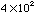has one significant figure.

• Exact numbers have an infinite number of significant digits. For example, if there are two oranges on a table, then the number of oranges is 2.000... . Defined numbers are also like this. For example, the number of centimeters per inch (2.54) has an infinite number of significant digits, as does the speed of light (299792458 m/s).

There are also specific rules for how to consistently express the uncertainty associated with a number. In general, the last significant figure in any result should be of the same order of magnitude (i.e.. in the same decimal position) as the uncertainty. Also, the uncertainty should be rounded to one or two significant figures. Always work out the uncertainty after finding the number of significant figures for the actual measurement.

For example,

9.82 +/- 0.02
10.0 +/- 1.5
4 +/- 1

The following numbers are all incorrect.

9.82 +/- 0.02385 is wrong but 9.82 +/- 0.02 is fine
10.0 +/- 2 is wrong but 10.0 +/- 2.0 is fine
4 +/- 0.5 is wrong but 4.0 +/- 0.5 is fine

In practice, when doing mathematical calculations, it is a good idea to keep one more digit than is significant to reduce rounding errors. But in the end, the answer must be expressed with only the proper number of significant figures. After addition or subtraction, the result is significant only to the place determined by the largest last significant place in the original numbers. For example,

89.332 + 1.1 = 90.432

should be rounded to get 90.4 (the tenths place is the last significant place in 1.1). After multiplication or division, the number of significant figures in the result is determined by the original number with the smallest number of significant figures. For example,

(2.80) (4.5039) = 12.61092

should be rounded off to 12.6 (three significant figures like 2.80).

Refer to any good introductory chemistry textbook for an explanation of the methodology for working out significant figures.

## The Idea of Error

The concept of error needs to be well understood. What is and what is not meant by "error"?

A measurement may be made of a quantity which has an accepted value which can be looked up in a handbook (e.g.. the density of brass). The difference between the measurement and the accepted value is not what is meant by error. Such accepted values are not "right" answers. They are just measurements made by other people which have errors associated with them as well.

Nor does error mean "blunder." Reading a scale backwards, misunderstanding what you are doing or elbowing your lab partner's measuring apparatus are blunders which can be caught and should simply be disregarded.

Obviously, it cannot be determined exactly how far off a measurement is; if this could be done, it would be possible to just give a more accurate, corrected value.

Error, then, has to do with uncertainty in measurements that nothing can be done about. If a measurement is repeated, the values obtained will differ and none of the results can be preferred over the others. Although it is not possible to do anything about such error, it can be characterized. For instance, the repeated measurements may cluster tightly together or they may spread widely. This pattern can be analyzed systematically.

## Classification of Error

Generally, errors can be divided into two broad and rough but useful classes: systematic and random.

Systematic errors are errors which tend to shift all measurements in a systematic way so their mean value is displaced. This may be due to such things as incorrect calibration of equipment, consistently improper use of equipment or failure to properly account for some effect. In a sense, a systematic error is rather like a blunder and large systematic errors can and must be eliminated in a good experiment. But small systematic errors will always be present. For instance, no instrument can ever be calibrated perfectly.

Other sources of systematic errors are external effects which can change the results of the experiment, but for which the corrections are not well known. In science, the reasons why several independent confirmations of experimental results are often required (especially using different techniques) is because different apparatus at different places may be affected by different systematic effects. Aside from making mistakes (such as thinking one is using the x10 scale, and actually using the x100 scale), the reason why experiments sometimes yield results which may be far outside the quoted errors is because of systematic effects which were not accounted for.

Random errors are errors which fluctuate from one measurement to the next. They yield results distributed about some mean value. They can occur for a variety of reasons.

• They may occur due to lack of sensitivity. For a sufficiently a small change an instrument may not be able to respond to it or to indicate it or the observer may not be able to discern it.

• They may occur due to noise. There may be extraneous disturbances which cannot be taken into account.

• They may be due to imprecise definition.

• They may also occur due to statistical processes such as the roll of dice.

Random errors displace measurements in an arbitrary direction whereas systematic errors displace measurements in a single direction. Some systematic error can be substantially eliminated (or properly taken into account). Random errors are unavoidable and must be lived with.

Many times you will find results quoted with two errors. The first error quoted is usually the random error, and the second is called the systematic error. If only one error is quoted, then the errors from all sources are added together. (In quadrature as described in the section on propagation of errors.)

A good example of "random error" is the statistical error associated with sampling or counting. For example, consider radioactive decay which occurs randomly at a some (average) rate. If a sample has, on average, 1000 radioactive decays per second then the expected number of decays in 5 seconds would be 5000. A particular measurement in a 5 second interval will, of course, vary from this average but it will generally yield a value within 5000 +/- . Behavior like this, where the error,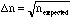, (1)

is called a Poisson statistical process. Typically if one does not know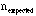it is assumed that,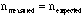,

in order to estimate this error.

### A. Mean Value

Suppose an experiment were repeated many, say N, times to get,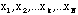,

N measurements of the same quantity, x. If the errors were random then the errors in these results would differ in sign and magnitude. So if the average or mean value of our measurements were calculated,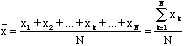, (2)

some of the random variations could be expected to cancel out with others in the sum. This is the best that can be done to deal with random errors: repeat the measurement many times, varying as many "irrelevant" parameters as possible and use the average as the best estimate of the true value of x. (It should be pointed out that this estimate for a given N will differ from the limit as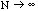the true mean value; though, of course, for larger N it will be closer to the limit.) In the case of the previous example: measure the height at different times of day, using different scales, different helpers to read the scale, etc.

Doing this should give a result with less error than any of the individual measurements. But it is obviously expensive, time consuming and tedious. So, eventually one must compromise and decide that the job is done. Nevertheless, repeating the experiment is the only way to gain confidence in and knowledge of its accuracy. In the process an estimate of the deviation of the measurements from the mean value can be obtained.

### B. Measuring Error

There are several different ways the distribution of the measured values of a repeated experiment such as discussed above can be specified.

• #### Maximum Error

The maximum and minimum values of the data set,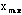and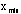, could be specified. In these terms, the quantity,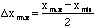, (3)

is the maximum error. And virtually no measurements should ever fall outside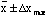.

• #### Probable Error

The probable error,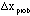, specifies the range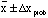which contains 50% of the measured values.

• #### Average Deviation

The average deviation is the average of the deviations from the mean,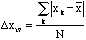. (4)

For a Gaussian distribution of the data, about 58% will lie within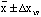.

• #### Standard Deviation

For the data to have a Gaussian distribution means that the probability of obtaining the result x is,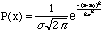, (5)

where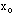is most probable value and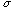, which is called the standard deviation, determines the width of the distribution. Because of the law of large numbers this assumption will tend to be valid for random errors. And so it is common practice to quote error in terms of the standard deviation of a Gaussian distribution fit to the observed data distribution. This is the way you should quote error in your reports.

It is just as wrong to indicate an error which is too large as one which is too small. In the measurement of the height of a person, we would reasonably expect the error to be +/-1/4" if a careful job was done, and maybe +/-3/4" if we did a hurried sample measurement. Certainly saying that a person's height is 5' 8.250"+/-0.002" is ridiculous (a single jump will compress your spine more than this) but saying that a person's height is 5' 8"+/- 6" implies that we have, at best, made a very rough estimate!

### C. Standard Deviation

The mean is the most probable value of a Gaussian distribution. In terms of the mean, the standard deviation of any distribution is,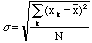. (6)

The quantity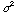, the square of the standard deviation, is called the variance. The best estimate of the true standard deviation is,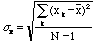. (7)

The reason why we divide by N to get the best estimate of the mean and only by N-1 for the best estimate of the standard deviation needs to be explained. The true mean value of x is not being used to calculate the variance, but only the average of the measurements as the best estimate of it. Thus,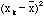as calculated is always a little bit smaller than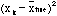, the quantity really wanted. In the theory of probability (that is, using the assumption that the data has a Gaussian distribution), it can be shown that this underestimate is corrected by using N-1 instead of N.

If one made one more measurement of x then (this is also a property of a Gaussian distribution) it would have some 68% probability of lying within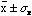. Note that this means that about 30% of all experiments will disagree with the accepted value by more than one standard deviation!

However, we are also interested in the error of the mean, which is smaller than sx if there were several measurements. An exact calculation yields,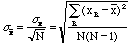, (8)

for the standard error of the mean. This means that, for example, if there were 20 measurements, the error on the mean itself would be = 4.47 times smaller then the error of each measurement. The number to report for this series of N measurements of x is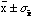where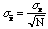. The meaning of this is that if the N measurements of x were repeated there would be a 68% probability the new mean value of would lie within(that is between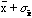and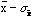). Note that this also means that there is a 32% probability that it will fall outside of this range. This means that out of 100 experiments of this type, on the average, 32 experiments will obtain a value which is outside the standard errors.

For a Gaussian distribution there is a 5% probability that the true value is outside of the range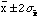, i.e. twice the standard error, and only a 0.3% chance that it is outside the range of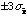.

## Examples

Suppose the number of cosmic ray particles passing through some detecting device every hour is measured nine times and the results are those in the following table.

Thus we have = 900/9 = 100 and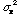= 1500/8 = 188 or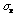= 14. Then the probability that one more measurement of x will lie within 100 +/- 14 is 68%.

The value to be reported for this series of measurements is 100+/-(14/3) or 100 +/- 5. If one were to make another series of nine measurements of x there would be a 68% probability the new mean would lie within the range 100 +/- 5.

Random counting processes like this example obey a Poisson distribution for which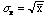. So one would expect the value ofto be 10. This is somewhat less than the value of 14 obtained above; indicating either the process is not quite random or, what is more likely, more measurements are needed.

```i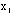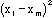------------------------------------------
1             80            400
2             95            25
3             100           0
4             110           100
5             90            100
6             115           225
7             85            225
8             120           400
9             105           25
S             900           1500
------------------------------------------

```

The same error analysis can be used for any set of repeated measurements whether they arise from random processes or not. For example in the Atwood's machine experiment to measure g you are asked to measure time five times for a given distance of fall s. The mean value of the time is,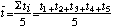, (9)

and the standard error of the mean is,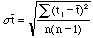, (10)

where n = 5.

For the distance measurement you will have to estimate [[Delta]]s, the precision with which you can measure the drop distance (probably of the order of 2-3 mm).

## Propagation of Errors

Frequently, the result of an experiment will not be measured directly. Rather, it will be calculated from several measured physical quantities (each of which has a mean value and an error). What is the resulting error in the final result of such an experiment?

For instance, what is the error in Z = A + B where A and B are two measured quantities with errors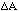and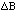respectively?

A first thought might be that the error in Z would be just the sum of the errors in A and B. After all,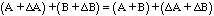(11)

and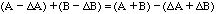. (12)

But this assumes that, when combined, the errors in A and B have the same sign and maximum magnitude; that is that they always combine in the worst possible way. This could only happen if the errors in the two variables were perfectly correlated, (i.e.. if the two variables were not really independent).

If the variables are independent then sometimes the error in one variable will happen to cancel out some of the error in the other and so, on the average, the error in Z will be less than the sum of the errors in its parts. A reasonable way to try to take this into account is to treat the perturbations in Z produced by perturbations in its parts as if they were "perpendicular" and added according to the Pythagorean theorem,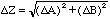. (13)

That is, if A = (100 +/- 3) and B = (6 +/- 4) then Z = (106 +/- 5) since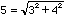.

This idea can be used to derive a general rule. Suppose there are two measurements, A and B, and the final result is Z = F(A, B) for some function F. If A is perturbed bythen Z will be perturbed by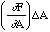where (the partial derivative) [[partialdiff]]F/[[partialdiff]]A is the derivative of F with respect to A with B held constant. Similarly the perturbation in Z due to a perturbation in B is,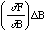.

Combining these by the Pythagorean theorem yields, (14)

In the example of Z = A + B considered above,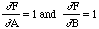,

so this gives the same result as before. Similarly if Z = A - B then,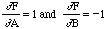,

which also gives the same result. Errors combine in the same way for both addition and subtraction. However, if Z = AB then,,

so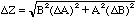, (15)

Thus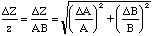, (16)

or the fractional error in Z is the square root of the sum of the squares of the fractional errors in its parts. (You should be able to verify that the result is the same for division as it is for multiplication.) For example,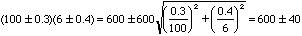.

It should be noted that since the above applies only when the two measured quantities are independent of each other it does not apply when, for example, one physical quantity is measured and what is required is its square. If Z = A2 then the perturbation in Z due to a perturbation in A is,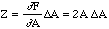. (17)

Thus, in this case,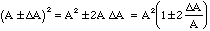(18)

and not A2 (1 +/-/A) as would be obtained by misapplying the rule for independent variables. For example,

(10 +/- 1)2 = 100 +/- 20 and not 100 +/- 14.

If a variable Z depends on (one or) two variables (A and B) which have independent errors (and) then the rule for calculating the error in Z is tabulated in following table for a variety of simple relationships. These rules may be compounded for more complicated situations.

```Relation between Z      Relation between errors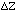and(A,B)                 and (,)
----------------------------------------------------------------
1      Z = A + B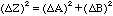2      Z = A - B3      Z = AB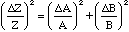4      Z = A/B5      Z = An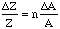6      Z =  ln A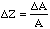7      Z  =  eA----------------------------------------------------------------
```

## References

1. Taylor, John R. An Introduction to Error Analysis: The Study of Uncertainties if Physical Measurements. University Science Books, 1982.

2. P.V. Bork, H. Grote, D. Notz, M. Regler. Data Analysis Techniques in High Energy Physics Experiments. Cambridge University Press, 1993.

Send comments, questions and/or suggestions via email to wolfs@nsrl.rochester.edu.